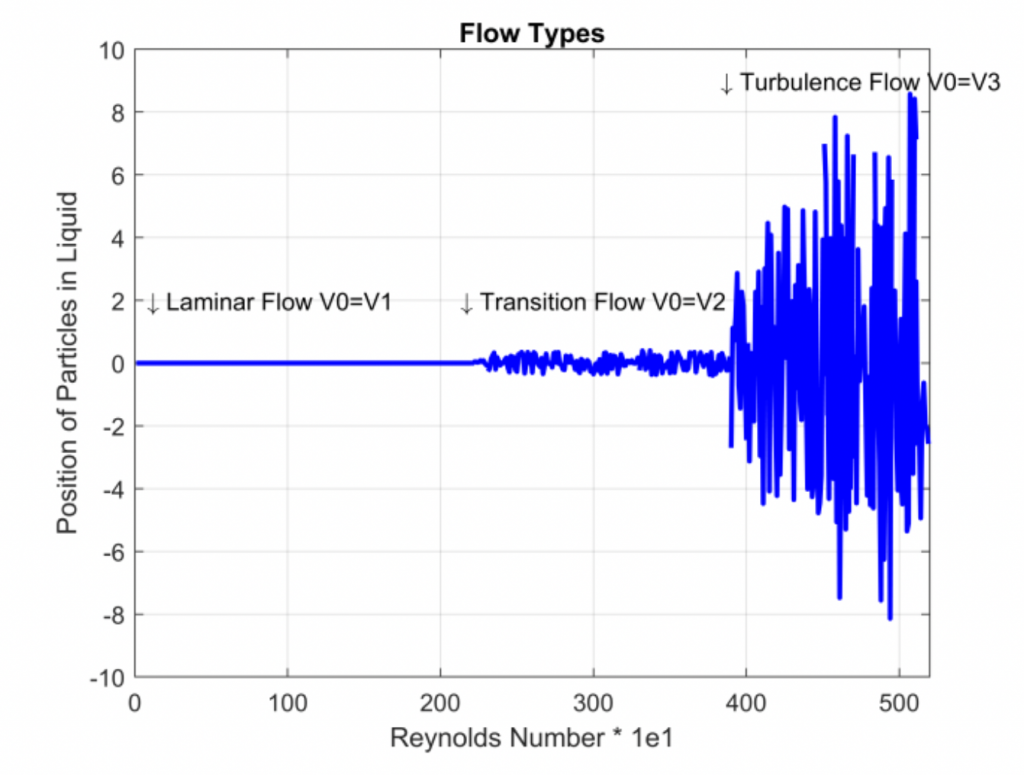## Simulation of Flow(Laminar, Turbulence)

In a turbulent flow, the fluid velocity at a fixed point varies with time in an almost random manner. As shown in the Figure 5.1. In other words, the velocity at a constant time t varies almost randomly with the position. Secondly, Matlab 2020 used for estimated simulation. In Equation 3.1, simulations were carried out keeping all data constant. (ceteris paribus) except flow velocity(V).Fluids geometry(laminar, transition, turbulence)

Firstly, it observed that the change in the velocity of the flow in the initial conditions causes changes in the response of the system. If Reynolds number <2300 is laminar flow. If 2300<Re<400 is transition flow. Similarly, if 4000<Re is turbulence flow.

As we can see in figure 5.1 and figure 5.2, the behaviour of the system depends on the initial conditions. This is called deterministic chaos. Deterministic chaos is an irregular behaviour sensitive to initial conditions. Any change (however small) in the initial state of the system leads to a completely different development of the system after some time.

Sensitive dependence on initial conditions (a property noted by Poincare, Birkhoff, and even Turing). Starting from very close initial conditions a chaotic system very rapidly moves to different states. Besides, as we can see in figure 5.3 and figure 5.4. After a while the system becomes unpredictable and turns into a complex structure. In conclusion, we call turbulence flow as Deterministic chaos.

## Conclusion

Firstly, this report shows that complex dynamics systems in fluids mechanics exist in many areas in our lives. One of them is turbulent flow in fluid mechanics. Secondly, it showed that it depends on the initial state, and the flow of turbulence was determined to be deterministic chaos. This and other reasons cause the turbulence flow to house unsolvable physics, geometry, and energy that are still unknown today.

## References

1.  (On the influence of memory on complex dynamics of evolutionary oligopoly models，2020)
2.  (How macroscopic laws describe complex dynamics. Asymptomatic population and CoviD-19 spreading， 2020)
3.  (Study of complex dynamics in DC- DC boost converter with dSPAC. Based real time controller，2020)
4.  (Refinement of previous hypotheses concerning the local structure of turbulence in viscous incompressible fluid，1961)
5.  (Large-eddy simulation: Past, present and the future，2014)

First Part :